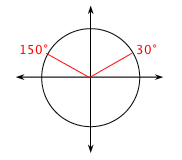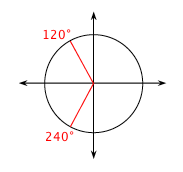### Home > INT3 > Chapter 12 > Lesson 12.1.3 > Problem12-37

12-37.

Solve each equation for $0^\circ\leθ<360^\circ$, or if you prefer, $0\leθ<2\pi$. You may need your calculator, but remember that your calculator only gives one value.

1. $\sin(θ)=0.5$

Use inverse sine on your calculator to find one solution.
$\sin^{−1}(0.5)=θ$

It should tell you the answer is $30^\circ$.

Draw a unit circle to find the other angle that makes $\sin(θ)=0.5$. The sine function is positive in quadrants I and II, so draw a reference angle of $30^\circ$ in each quadrant.2. $\cos(θ)=-0.5$

Follow the steps in part (a).3. $4\tan(θ)-4=0$

First solve the equation for $\tan(θ)$.
$\tan(θ)=1$.

Follow the steps in part (a).

$45^\circ$ and $225^\circ$